# Methods and formulas for the model summary in Fit Binary Logistic Model

Select the method or formula of your choice.

## Deviance R2

The deviance R2 indicates how much variation in the response is explained by the model. The higher the R2, the better the model fits your data. The formula is:

### Notation

TermDescription
DEError Deviance
DTTotal Deviance

The adjusted deviance R2 accounts for the number of predictors in your model and is useful for comparing models with different numbers of predictors. The formula is:

### Notation

TermDescription
R2the deviance R2
pthe regression degrees of freedom
Φ1, for binomial and Poisson models
DTthe total deviance

While the calculations for adjusted deviance R2 can produce negative values, Minitab displays zero for these cases.

## Akaike Information Criterion (AIC)

Use this statistic to compare different models. The smaller AIC is, the better the model fits the data.

The log-likelihood functions are parameterized in terms of the means. The general form of the functions follow:

The general form of the individual contributions follows:

The specific form of the individual contributions depends on the model.

 Model li BinomialPoisson### Notation

TermDescription
pthe regression degrees of freedom
Lcthe log-likelihood of the current model
yithe number of events for the ith row
mithe number of trials for the ith rowthe estimated mean response of the ith row

## AICc (Akaike's Corrected Information Criterion)

AICc is not calculated when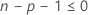.

### Notation

TermDescription
pthe number of coefficients in the model, including the constant
nthe number of rows in the data with no missing data

## BIC (Bayesian Information Criterion)

### Notation

TermDescription
pthe number of coefficients in the model, not counting the constant
nthe number of rows in the data with no missing data

## Test deviance R2

The test deviance R2 indicates how much of the variation in the response of the test data set the model explains. The higher the value, the better the model fits the test data.

### Formula

The following equation gives the formula for the test deviance R2:

where the following equation represents the error deviance:

The formula for the total deviance, DT(Test), depends on the form of the model.
Binary logistic
where for models with an intercept term,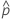has the following definition:
For models without an intercept term, use the inverse of the link function at 0. The values for the link functions in Minitab follow:= 0.5.= 0.5.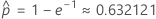.
Poisson
where for models with an intercept term
For models without an intercept term,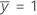.

### Notation

TermDescription
N(Test)the number of rows in the test data set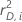the squared deviance residuals
yithe number of events for the ith row in the test data set
mithe number of trials for the ith row in the test data set
DE(Test)the error deviance for the test data set
DT(Test)the total deviance for the test data set

## K-fold Deviance R2

The k-fold deviance R2 indicates how much of the variation in the response of the validation data set the model explains. The higher the value, the better the model fits the test data.

Where

and DT is the total deviance.

### Notation

TermDescription
Knumber of folds
njsample size of fold j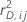cross validated deviance residual for the ith row of fold j

## Area under ROC curve

### Formula

The area under the curve is the summation of areas of trapezoids:

where k is the number of distinct event probabilities and (x0, y0) is the point (0, 0).

To compute the area for a curve from a test data set or from cross-validated data, use the points from the corresponding curve.

For example, suppose we have four distinct event probabilities with the following coordinates on the ROC curve:
x (false positive rate) y (true positive rate)
0.0923 0.3051
0.4154 0.7288
0.7538 0.9322
1 1
Then the area under the ROC curve is given by the following calculation: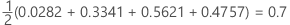### Notation

TermDescription
TRP true positive rate
FPR false positive rate
TPtrue positive, events that were correctly assessed
P number of actual positive events
FPtrue negative, nonevents that were correctly assessed
N number of actual negative events
FNRfalse negative rate
TNRtrue negative rate